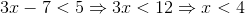## Example Questions

### Example Question #1 : Inequalities

Given that x = 2 and y = 4, how much less is the value of  2x2 –  2y than the value of  2y2 –  2x ?

12

52

2

28

28

Explanation:

First, we solve each expression by plugging in the given values for x and y:

2(22) – 2(4) = 8 – 8 = 0

2(42) – 2(2) = 32 – 4 = 28

Then we find the difference between the first and second expressions’ values:

28 – 0 = 28

### Example Question #2 : Inequalities

Solve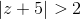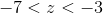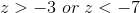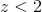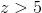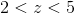Explanation:

Absolute value problems are broken into two inequalities: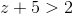and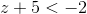.  Each inequality is solved separately to get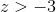and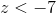.  Graphing each inequality shows that the correct answer is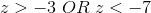.

### Example Question #3 : Inequalities

Which of the following inequalities defines the solution set to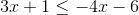?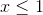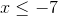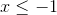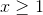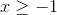Explanation:

First, move thes to one side.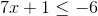Subtract by 1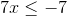Divide both sides by 7.### Example Question #1 : Inequalities

The cost, in cents, of manufacturingpencils is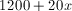, where 1200 is the number of cents required to run the factory regardless of the number of pencils made, and 20 represents the per-unit cost, in cents, of making each pencil. The pencils sell for 50 cents each. What number of pencils would need to be sold so that the revenue received is at least equal to the manufacturing cost?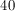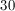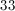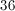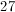Explanation:

If each pencil sells at 50 cents,pencils will sell at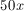. The smallest value ofsuch that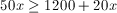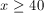### Example Question #4 : Inequalities

Solve the following inequality: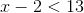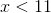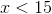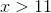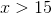Explanation:

To solve an inequality with subtraction, simply solve it is as an equation.

The goal is to isolate the variable on one side with all other constants on the other side. Perform the opposite operation to manipulate the inequality.

In this case add two to each side.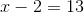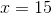### Example Question #5 : Inequalities

Solve the following inequality: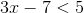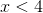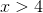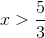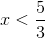Explanation:

To solve, simply treat it as an equation.

This means you want to isolate the variable on one side and move all other constants to the other side through opposite operation manipulation.

Remember, you only flip the inequality sign if you multiply or divide by a negative number.

Thus,